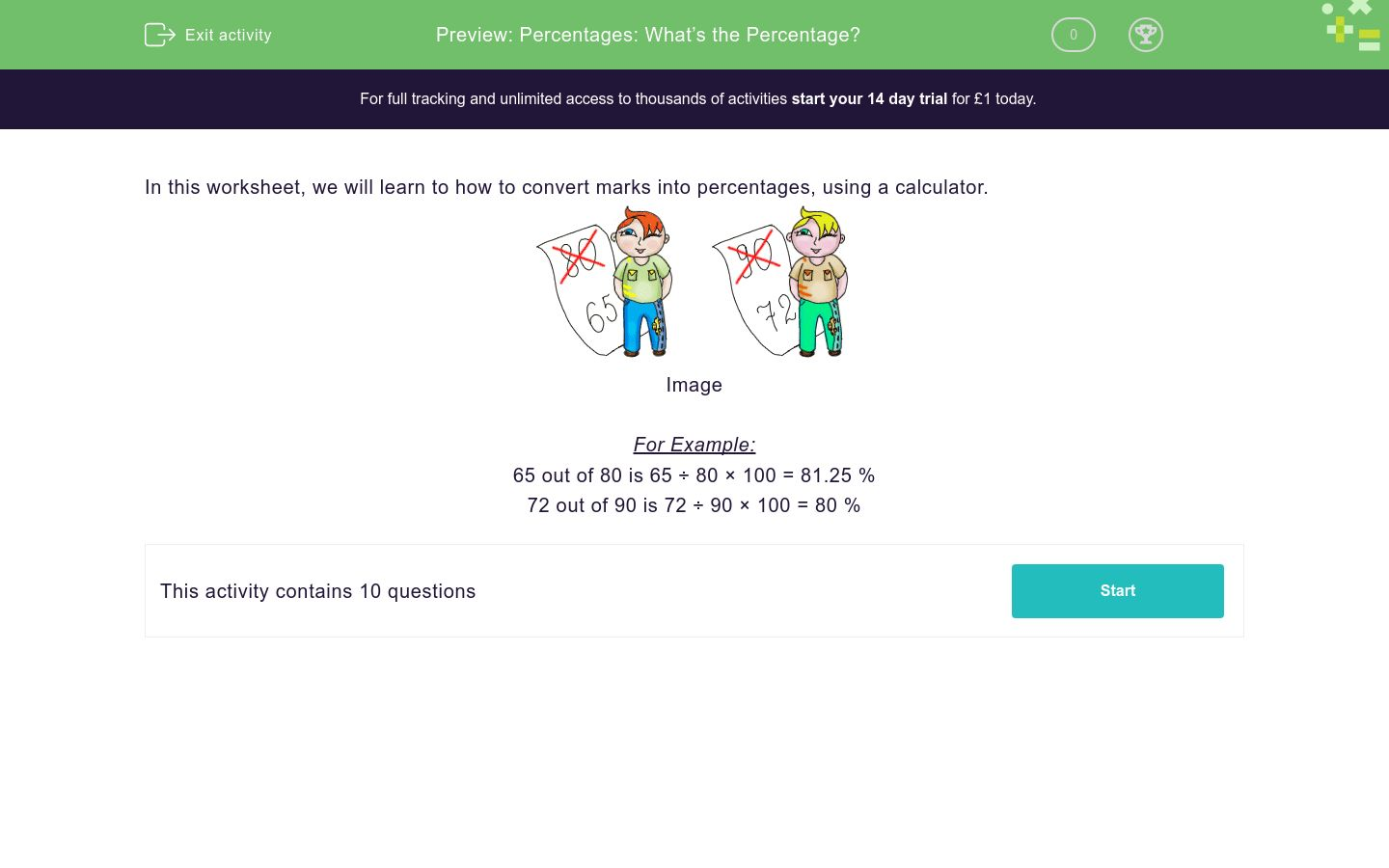# Percentages: What’s the Percentage?

In this worksheet, students change marks expressed as fractions into percentages. They can use a calculator to check their workings.Key stage:  KS 2

Curriculum topic:   Ratio and Proportion

Curriculum subtopic:   Solve Percentage Problems

Difficulty level:### QUESTION 1 of 10

In this worksheet, we will learn to how to convert marks into percentages, using a calculator.For Example:

65 out of 80 is 65 ÷ 80 × 100 = 81.25 %

72 out of 90 is 72 ÷ 90 × 100 = 80 %

Work out these marks as percentages, rounding off your answer to 2 decimal places.

80 out of 89 is _________ %

Work out these marks as percentages, rounding off your answer to 2 decimal places.

87 out of 98 is _________ %

Work out these marks as percentages, rounding off your answer to 2 decimal places.

61 out of 73 is _________ %

Work out these marks as percentages, rounding off your answer to 2 decimal places.

30 out of 43 is _________ %

Work out these marks as percentages, rounding off your answer to 2 decimal places.

47 out of 84 is _________ %

Work out these marks as percentages, rounding off your answer to 2 decimal places.

98 out of 99 is _________ %

Work out these marks as percentages, rounding off your answer to 2 decimal places.

62 out of 72 is _________ %

Work out these marks as percentages, rounding off your answer to 2 decimal places.

32 out of 45 is _________ %

Work out these marks as percentages, rounding off your answer to 2 decimal places.

70 out of 99 is _________ %

Work out these marks as percentages, rounding off your answer to 2 decimal places.

77 out of 97 is _________ %

• Question 1

Work out these marks as percentages, rounding off your answer to 2 decimal places.

80 out of 89 is _________ %

89.89
EDDIE SAYS
Use your calculator to work out 80 ÷ 89 x 100
• Question 2

Work out these marks as percentages, rounding off your answer to 2 decimal places.

87 out of 98 is _________ %

88.78
• Question 3

Work out these marks as percentages, rounding off your answer to 2 decimal places.

61 out of 73 is _________ %

83.56
• Question 4

Work out these marks as percentages, rounding off your answer to 2 decimal places.

30 out of 43 is _________ %

69.77
• Question 5

Work out these marks as percentages, rounding off your answer to 2 decimal places.

47 out of 84 is _________ %

55.95
• Question 6

Work out these marks as percentages, rounding off your answer to 2 decimal places.

98 out of 99 is _________ %

98.99
• Question 7

Work out these marks as percentages, rounding off your answer to 2 decimal places.

62 out of 72 is _________ %

86.11
• Question 8

Work out these marks as percentages, rounding off your answer to 2 decimal places.

32 out of 45 is _________ %

71.11
• Question 9

Work out these marks as percentages, rounding off your answer to 2 decimal places.

70 out of 99 is _________ %

70.71
• Question 10

Work out these marks as percentages, rounding off your answer to 2 decimal places.

77 out of 97 is _________ %

79.38
---- OR ----

Sign up for a £1 trial so you can track and measure your child's progress on this activity.

### What is EdPlace?

We're your National Curriculum aligned online education content provider helping each child succeed in English, maths and science from year 1 to GCSE. With an EdPlace account you’ll be able to track and measure progress, helping each child achieve their best. We build confidence and attainment by personalising each child’s learning at a level that suits them.

Get started